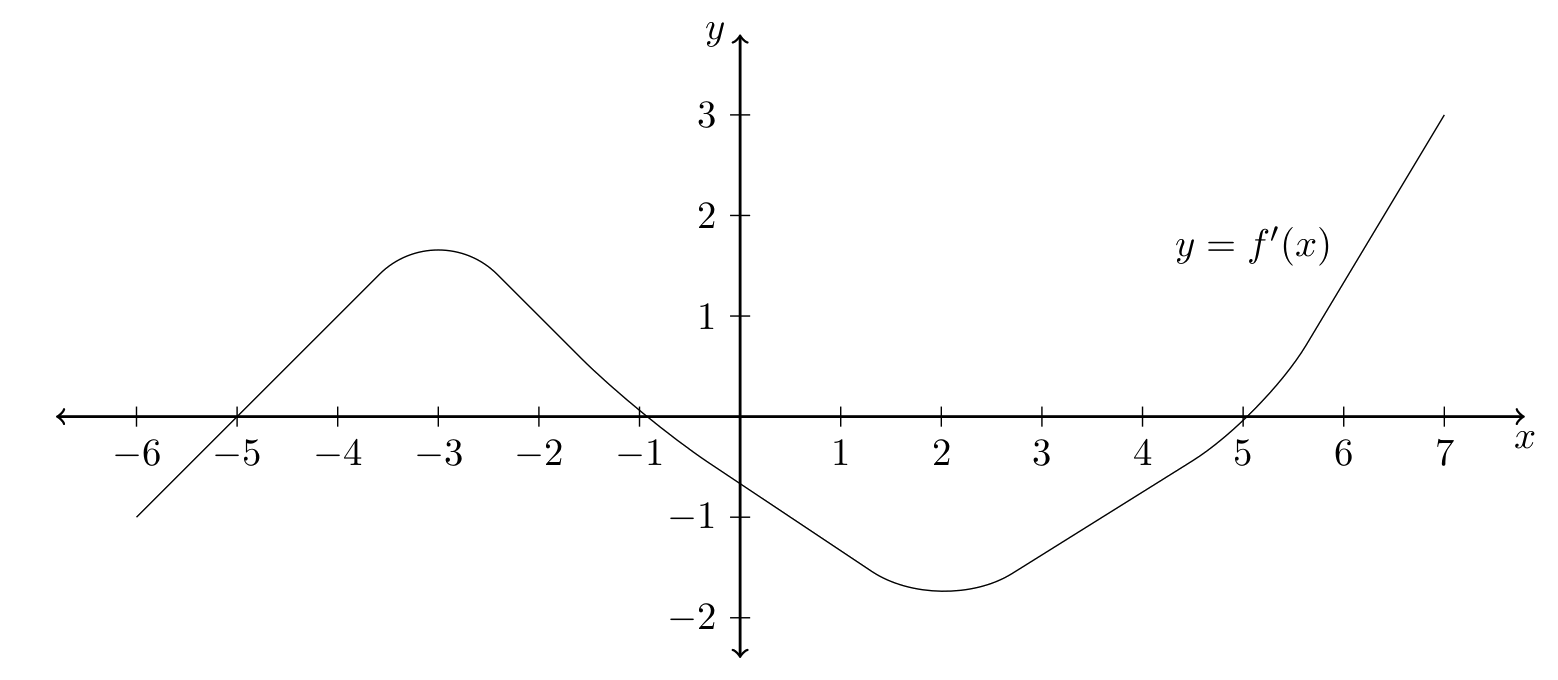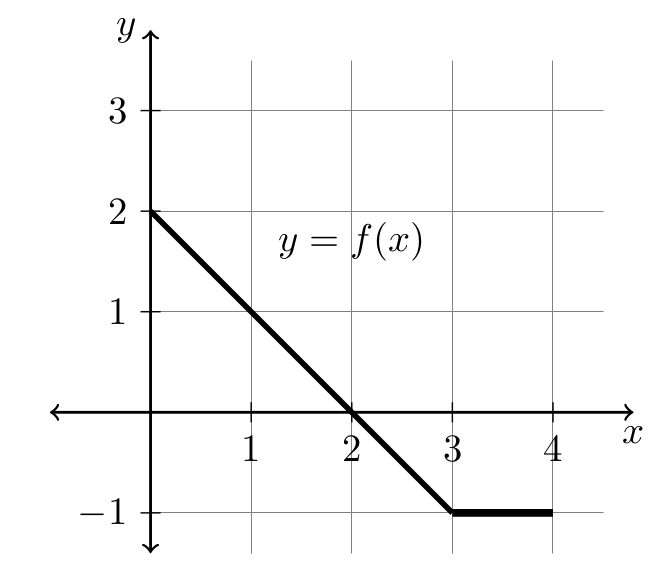MTH2205 Home

## MTH 2205: Final Practice 2 (Part 1: No calculators)

Problem 1. Find the absolute minimum value of $$f(x)=\frac{2x}{x^2+1}$$ on the interval $$0\leq x\leq 2$$.

(A) $$-1$$ $$\quad\quad$$ (B) $$0$$ $$\quad\quad$$ (C) $$1$$ $$\quad\quad$$ (D) $$\frac45$$ $$\quad\quad$$ (E) $$2$$

Problem 2. The functions $$f(x)$$ and $$f^{-1}(x)$$ are inverses of one another, where $$f(-1)=5$$, $$f'(5)=7$$, and $$f'(-1)=4$$. Then $$\left(f^{-1}\right)'(5)$$ is

(A) $$-4$$ $$\quad\quad$$ (B) $$\frac15$$ $$\quad\quad$$ (C) $$\frac17$$ $$\quad\quad$$ (D) $$\frac14$$ $$\quad\quad$$ (E) $$-5$$

Problem 3. The cost of producing $$x$$ items is $$C(x)=2x^3-20x^2+100x$$. Find the value of $$x$$ that minimizes the average cost.

(A) $$5$$ $$\quad\quad$$ (B) $$6$$ $$\quad\quad$$ (C) $$3$$ $$\quad\quad$$ (D) $$8$$ $$\quad\quad$$ (E) $$2$$

Problem 4. Solve for $$x$$: $$\left(\frac13\right)^{1-x}=9$$.

(A) $$-2$$ $$\quad\quad$$ (B) $$0$$ $$\quad\quad$$ (C) $$-1$$ $$\quad\quad$$ (D) $$2$$ $$\quad\quad$$ (E) $$3$$

Problem 5. Solve for $$x$$: $$\log_2(x)+\log_2(x-7)=3$$.

(A) $$x=1$$ and $$x=8$$ $$\quad\quad$$ (B) $$x=-1$$ only $$\quad\quad$$ (C) $$x=8$$ only $$\quad\quad$$
(D) $$x=3$$ and $$x=4$$ $$\quad\quad$$ (E) $$x=-1$$ and $$x=8$$

Problem 6. If $$f(x)=xe^{x^2+1}$$, then $$f'(2)$$ is

(A) $$9e^5$$ $$\quad\quad$$ (B) $$6e^5$$ $$\quad\quad$$ (C) $$2e^5$$ $$\quad\quad$$ (D) $$8e^5$$ $$\quad\quad$$ (E) $$e^4$$

Problem 7. $$\int_0^4\frac1{3x+1}\,dx=$$

(A) $$\frac1{13}$$ $$\quad\quad$$ (B) $$\frac1{13}\ln4$$ $$\quad\quad$$ (C) $$\frac1{3}\ln13$$ $$\quad\quad$$ (D) $$\frac14$$ $$\quad\quad$$ (E) $$\frac3{3x+1}$$

Problem 8. Find $$\frac{dy}{dx}$$ for $$y=\ln\left(\frac{\sqrt{x-5}}{4x^3+1}\right)$$.

(A) $$\frac{\sqrt{x-5}}{4x^3+1}$$ $$\quad\quad$$ (B) $$\frac1{2(x-5)}-\frac{12x^2}{4x^3+1}$$ $$\quad\quad$$ (C) $$\frac{4x^3+1}{\sqrt{x-5}}$$ $$\quad\quad$$
(D) $$e^{\sqrt{x-5}}-e^{4x^3+1}$$ $$\quad\quad$$ (E) $$\frac1{2\sqrt{x-5}}-\frac{12x^2}{4x^3+1}$$

Problem 9. If the second derivative of $$f(x)$$ is $$f''(x)=(3-x)(x^2-4)$$, on what interval(s) is $$f(x)$$ concave up?

(A) $$(-\infty,-2)$$ and (2,3) $$\quad\quad$$ (B) $$(-2,2)$$ $$\quad\quad$$ (C) $$(-\infty,\infty)$$ $$\quad\quad$$
(D) $$(2,\infty)$$ $$\quad\quad$$ (E) $$(-2,2)$$ and $$(3,\infty)$$

Problem 10. The graph of $$f'(x)$$, the derivative of $$f$$, is given below for $$-6\leq x\leq 7$$. On what intervals is the function, $$f(x)$$, increasing?(A) $$(-3,2)$$ $$\quad\quad$$ (B) $$(-3,7)$$ $$\quad\quad$$ (C) $$(-5,-1)$$ and $$(5,7)$$ $$\quad\quad$$
(D) $$(-6,-3)$$ and $$(2,7)$$ $$\quad\quad$$ (E) $$(-6,-5)$$ and $$(-1,5)$$

Problem 11. The graph of $$y=f(x)$$ is given below. Evaluate $$\int_0^4f(x)\,dx$$.(A) $$0.5$$ $$\quad\quad$$ (B) $$1.5$$ $$\quad\quad$$ (C) $$-0.5$$ $$\quad\quad$$ (D) $$3.5$$ $$\quad\quad$$ (E) $$-2.5$$

Problem 12. An object moving on a line has velocity given by $$v(t)=3t^2-4t+6$$, $$t\geq 0$$. At time $$t=1$$ the object's position is $$s(1)=2$$. Find $$s(t)$$, the object's position at any time $$t$$.

(A) $$s(t)=t^3-2t^2+6t+2$$ $$\quad\quad$$ (B) $$s(t)=6t-4$$ $$\quad\quad$$ (C) $$s(t)=t^3-2t^2+6t-3$$ $$\quad\quad$$
(D) $$s(t)=t^4-2t^3+6t^2-3$$ $$\quad\quad$$ (E) $$s(t)=5$$

Problem 13. Linearize $$f(x)=x^4$$ at $$x=2$$, and then use the linearization to approximate $$f(2.01)$$.

(A) $$16.01$$ $$\quad\quad$$ (B) $$16.24$$ $$\quad\quad$$ (C) $$18.24$$ $$\quad\quad$$ (D) $$16.32$$ $$\quad\quad$$ (E) $$16.04$$

Problem 14. If $$f(x)=\frac{4x-1}{2x+3}$$, then the inverse function $$f^{-1}(x)$$ is

(A) $$\frac{2x+3}{4x-1}$$ $$\quad\quad$$ (B) $$\frac{1-4x}{3-2x}$$ $$\quad\quad$$ (C) $$\frac{4-2x}{3x+1}$$ $$\quad\quad$$
(D) $$\frac{3x+1}{4-2x}$$ $$\quad\quad$$ (E) $$3x+1+\frac1{4-2x}$$

Problem 15. The function $$f(x)=4x^3+9x^2+6x-5$$ has a point of inflection at

(A) $$x=1$$ $$\quad\quad$$ (B) $$x=-\frac12$$ $$\quad\quad$$ (C) $$x=\frac14$$ $$\quad\quad$$
(D) $$x=-\frac34$$ $$\quad\quad$$ (E) $$x=-\frac12$$ and $$x=-1$$

Problem 16. Evaluate $$\displaystyle \int_1^4\left(3\sqrt x+\frac{4}{x^2}\right)\,dx$$

(A) $$6$$ $$\quad\quad$$ (B) $$7$$ $$\quad\quad$$ (C) $$18$$ $$\quad\quad$$ (D) $$10$$ $$\quad\quad$$ (E) $$17$$

Problem 17. Evaluate $$\displaystyle \sum_{i=1}^4i(4-i)$$

(A) $$0$$ $$\quad\quad$$ (B) $$-10$$ $$\quad\quad$$ (C) $$10$$ $$\quad\quad$$ (D) $$9$$ $$\quad\quad$$ (E) $$-9$$

Problem 18. $$\displaystyle \int \left(x^3+2x\right)^5\left(12x^2+8\right)\,dx$$

(A) $$\frac16\left(x^3+2x\right)^6+C$$ $$\quad\quad$$ (B) $$\frac16\left(x^3+2x\right)^6\cdot\frac12\left(12x^2+8\right)^2+C$$ $$\quad\quad$$ (C) $$\frac12\left(12x^2+8\right)^2+C$$ $$\quad\quad$$
(D) $$\frac23\left(x^3+2x\right)^6+C$$ $$\quad\quad$$ (E) $$\left(x^3+2x\right)^6+C$$

Problem 19. Solve the differential equation $$\frac{dy}{dx}=\frac{x^2}{y^2}$$, $$y\neq 0$$, with the initial condition $$y(0)=2$$.

(A) $$y=\sqrt{x^2+8}$$ $$\quad\quad$$ (B) $$y=x+8$$ $$\quad\quad$$ (C) $$y=\sqrt{x^3+1}$$ $$\quad\quad$$
(D) $$y=\sqrt{x^3+1}$$ $$\quad\quad$$ (E) $$y=\sqrt{x^3+8}$$

Problem 20. The demand equation is $$x+3p^2=1000$$, where $$p$$ is the price. Find the elasticity of demand if $$p=10$$.

(A) $$-\frac49$$ $$\quad\quad$$ (B) $$-\frac16$$ $$\quad\quad$$ (C) $$-2$$ $$\quad\quad$$ (D) $$-\frac67$$ $$\quad\quad$$ (E) $$-\frac58$$

Problem 21. The difference of one number $$x$$ and twice a second number $$y$$ is $$16$$. What is the minimum possible product of $$x$$ and $$y$$?

(A) $$-32$$ $$\quad\quad$$ (B) $$-24$$ $$\quad\quad$$ (C) $$80$$ $$\quad\quad$$ (D) $$12$$ $$\quad\quad$$ (E) $$-48$$

Problem 22. The function $$f(x)=2x^3+3x^2-36x$$ has a relative maximum at

(A) $$x=-3$$ $$\quad\quad$$ (B) $$x=-2$$ $$\quad\quad$$ (C) $$x=0$$ $$\quad\quad$$ (D) $$x=2$$ $$\quad\quad$$ (E) $$x=3$$

Problem 23. The approximate area bounded by $$y=5-x^2$$, the $$x$$-axis, $$x=-1$$ and $$x=2$$, using three rectangles of equal width and right hand endpoints is:

(A) $$9$$ $$\quad\quad$$ (B) $$10$$ $$\quad\quad$$ (C) $$11$$ $$\quad\quad$$ (D) $$12$$ $$\quad\quad$$ (E) $$13$$

Problem 24. If $$f(2)=7$$, $$f'(2)=0$$, and $$f''(2)=-5$$, then the point $$(2,7)$$ is

(A) a relative maximum $$\quad\quad$$ (B) a relative minimum $$\quad\quad$$ (C) a point of inflection $$\quad\quad$$
(D) a point of discontinuity $$\quad\quad$$ (E) none of the above

Problem 25. Find the average value of $$f(x)=4x-x^2$$ on the interval $$0\leq x\leq 2$$.

(A) $$\frac{16}3$$ $$\quad\quad$$ (B) $$2$$ $$\quad\quad$$ (C) $$4$$ $$\quad\quad$$ (D) $$8$$ $$\quad\quad$$ (E) $$\frac83$$